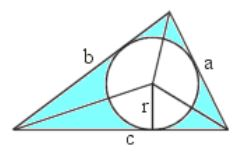Courses

# Theory - To illustrate that the Internal Bisectors of the Angles of a Triangle Concur at a Point Class 9 Notes | EduRev

## Mathematics (Maths) Class 9

Created by: Indu Gupta

## Class 9 : Theory - To illustrate that the Internal Bisectors of the Angles of a Triangle Concur at a Point Class 9 Notes | EduRev

The document Theory - To illustrate that the Internal Bisectors of the Angles of a Triangle Concur at a Point Class 9 Notes | EduRev is a part of the Class 9 Course Mathematics (Maths) Class 9.
All you need of Class 9 at this link: Class 9

Objective:

To illustrate that the internal bisectors of the angles of a triangle concur at a point (called the incentre), which always lies inside the triangle.

Related terms

• Incentre- Incentre of a triangle is defined as the point of intersection of the internal bisectors of a triangle. By internal bisectors, we mean the angle bisectors of interior angles of a triangle. Since there are three interior angles in a triangle, there must be three internal bisectors. The intersection point of all three internal bisectors is known as incentre of a circle.

• Incircle-  In geometry, the incircle or inscribed circle of a triangle is the largest circle contained in the triangle; it touches (is tangent to) the three sides. The center of the incircle is called the triangle's incentre.Properties:

• The incentre is one of the triangle's points of concurrency formed by the intersection of the triangle's three angle bisectors.

• These three angle bisectors are always concurrent and always meet in the triangle's interior (unlike the orthocenter which may or may not intersect in the interior). The incentre is the center of the incircle. The incentre is the one point in the triangle whose distances to the sides are equal.

• If the triangle is obtuse, then the incentre is located in the triangle's interior.

• If the triangle is acute, then the incentre is also located in the triangle's interior.

• If the triangle is right, then the incentre is also located in the triangle's interior.

## Mathematics (Maths) Class 9

190 videos|232 docs|82 tests

,

,

,

,

,

,

,

,

,

,

,

,

,

,

,

,

,

,

,

,

,

;